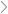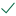Questions & AnswersMicroeconomicsFind  b elow some costs of production by a perfectly competitive...

QuestionAnswered step-by-step

# Find  b elow some costs of production by a perfectly competitive...

1. Find below some costs of production by a perfectly competitive Company, AB Metals:

Quantity                  Total Fixed Cost            Total Variable Cost

0                                          50                                0

1                                          50                                50

2                                          50                                70

3                                          50                                90

4                                          50                                140

5                                          50                                200

6                                          50                                360

a. Calculate the following: marginal cost, average total cost average variable cost, average fixed cost, at every level of production.

b. The price of a piston is 50. What is the profit or loss if the company decides to shut down operations? Explain.Solved by verified expert10,000 step-by-step explanations